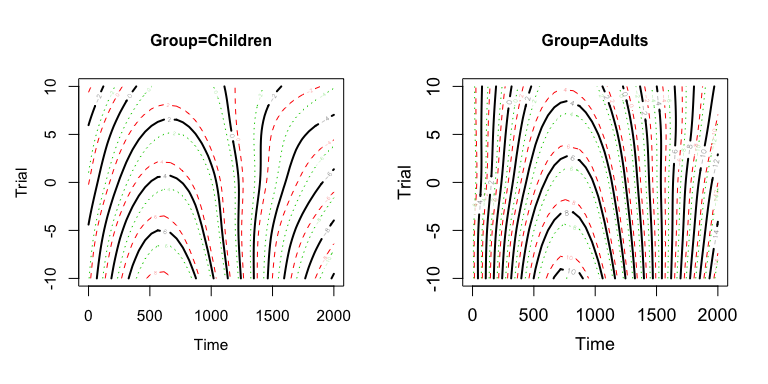# Introduction

The package `itsadug` (http://github.com/vr-vr/itsadug) includes several plot functions to visualize the estimates of Generalized Additive (Mixed) Models (GAMM) implemented using the package `mgcv` (Wood 2006; 2011). This paper presents a short overview of:

• the available plot functions in the packages `mgcv` and `itsadug`;

• the functions to retrieve predicted values from a GAMM, and how these could be used to create custom plots;

• the plot functions for inspection of the residuals.

## Example GAMM model

The code below was used to fit a GAMM model `m1` to the data set `simdat` from the package `itsadug`. The data set `simdat` is simulated time series data with arbitrary predictors. We use the interaction between the predictors Time and Trial to illustrate the various functions that are available for visualizing nonlinear interactions.

``library(itsadug)``
``````## Loading required package: mgcv
## This is mgcv 1.8-3. For overview type 'help("mgcv-package")'.
``````data(simdat)

# For illustration purposes, we build a GAMM model
# with a nonlinear interaction, two groups, and
# random wiggly smooths for Subjects:
m1 <- bam(Y ~ Group + te(Time, Trial, by=Group)
+ s(Time, Subject, bs='fs', m=1),
data=simdat)``````

The function `gamtabs` converts the summary quickly in a Latex table or in a HTML table (specify `type="html"`), which could be included in a `knitr` file.

``gamtabs(m1, caption="Summaty of m1", comment=FALSE, type='html')``
 A. parametric coefficients Estimate Std. Error t-value p-value (Intercept) 1.7930 0.6466 2.7730 0.0056 GroupAdults 2.4421 0.9197 2.6552 0.0079 B. smooth terms edf Ref.df F-value p-value te(Time,Trial):GroupChildren 23.0273 23.1209 1579.9964 < 0.0001 te(Time,Trial):GroupAdults 23.3166 23.3935 1302.5649 < 0.0001 s(Time,Subject) 317.5683 322.0000 3291.1419 < 0.0001

# Nonlinear interactions

## 1. plot.gam() for partial effects

The default way to plot interactions is to use `mgcv`’s `plot.gam`. This function visualizes the partial effects, see Figure 1.

``````par(mfrow=c(1,2))
plot(m1, select=1, rug=FALSE,
main='Group=Children', cex.axis=1.5, cex.lab=1.5)
plot(m1, select=2, rug=FALSE,Figure 1. Partial effects surfaces `te(Time,Trial):GroupChildren` and `te(Time,Trial):GroupAdults` plotted with `plot.gam`.

Advantage and disadvantages of `plot.gam`:

• Confidence bands are plotted, which are useful for seeing whether effects are significant.

• Without colored background the contour plots are difficult to read. It is possible to plot a colored background using the argument `scheme=2`, but only `heat.colors` are possible.

• The use of `plot.gam` is limited to interactions with two (or less) continuous variables.

## 2. pvisgam() for partial effects

Alternatively one could use the function `pvisgam` from the package `itsadug` to visualize the partial effects. The function plots exactly the same surfaces as `plot.gam`, but visualizes the surface slightly different.

``````par(mfrow=c(1,2))
# Note: specify zlim when comparing two plots
pvisgam(m1, view=c("Time", "Trial"), select=1,
main='Group=Children', labcex=.8,
zlim=c(-15,15), print.summary=FALSE)
pvisgam(m1, view=c("Time", "Trial"), select=2,